Home > CCA2 > Chapter 12 > Lesson 12.2.2 > Problem12-122

12-122.
1. Solve for x, correct to three decimal places. Homework Help ✎

1. 27 = 6x

2. 27 =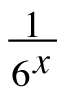3. 27 =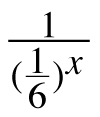4. Show that= 6x.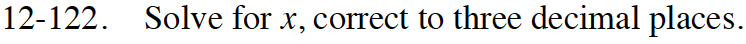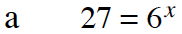How do you ''undo'' an exponent?
What do you have to do to both sides of the equation?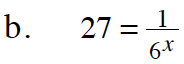Rearrange the equation so that the term withthe exponent is no longer in the denominator.

x ≈ −1.839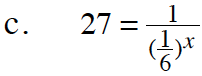This is the same as part (a). Why?

x ≈ 1.839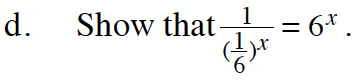This is similar to part (c), but you already have the answer!